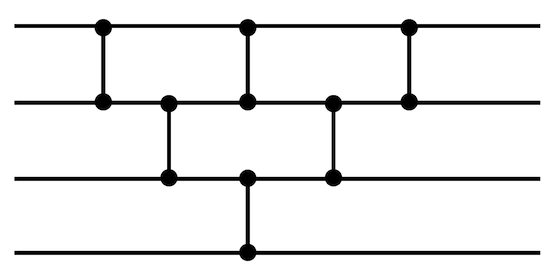# Sorting Networks using Higher-Order Functions of JavaScript Array

An implementation of a sorting algorithm usually uses one or more iterating loops in the form of for/while statement. With JavaScript and its powerful Array object, these loops can be avoided since Array’s higher-order functions are enough for iterating the array. One candidate for this technique is the implementation of sorting networks.

Before we start, let us do a quick refresh on some higher-order functions. In my previous blog post Searching using Array.prototype.reduce, there is an implementation of insertion sort without any loop statements at all. If we change the problem into sorting an array of numbers, the complete code will be:

``````function sort(entries) {
return Array.apply(undefined, Array(entries.length)).map(function () {
return entries.splice(entries.reduce(function (max, e, i) {
return e > max.value ? { index: i, value: e } : max;
}, { value: null }).index, 1).pop();
});
}

console.log(sort([14, 3, 77])); // [ 77, 14, 3 ]
``````

Like a typical insertion sort, the outer loop picks the largest value one at a time while the inner loop searches for the largest number in the working array. For the outer loop, `Array.apply(0, Array(N))` is the trick to generate a usable empty array, see my other blog post on Sequence using JavaScript Array. In the inner loop, `reduce` is used to locate the largest number as well as its index. The index is needed to yank that number out of the array. At the same time, the number is being stashed into the sorting result.

If you are still confused, try to deconstruct and debug the above code. When necessary, write the imperative version, possibly using the classical for loop, and compare both versions. It is quite useful to understand this properly to make it easier to follow the next part.

For the sorting network, the process involves two steps. The first step is to build the comparator network, the second is the actual sorting process via comparison and swap according to the constructed network. For the second step, the core operation is the following function (that acts like a comparator unit):

``````function compareswap(array, p, q) {
if (array[p] < array[q]) {
var temp = array[q];
array[q] = array[p];
array[p] = temp;
}
}
``````

As an illustration, if the array to be sorted has 3 numbers only, practically the sorting will be a series of the following steps:

``````compareswap(entries, , 1);
compareswap(entries, 1, 2);
compareswap(entries, , 1);
``````

For 4-number array, it will be like:

``````compareswap(entries, , 1);
compareswap(entries, 1, 2);
compareswap(entries, 2, 3);
compareswap(entries, , 1);
compareswap(entries, 1, 2);
compareswap(entries, , 1);
``````

If we draw the sequence, the sorting network annotation look like the following diagram. You probably can already see the pattern here, in particular if you relate it to the previous implementation of insertion sort. There is a few alternatives to this configuration of sorting networks such as odd-even mergesort, Bitonic, and many others.The comparator network simply formalizes this so that we can put every compare-and-swap action in a single loop. As long as we have the right network for the given array size, sorting is a matter of running:

``````function sort(network, entries) {
for (var i = ; i < network.length; ++i)
compareswap(entries, network[i], network[i] + 1)
}
``````

Quiz: what kind of sorting algorithm is that?

How to create the network? A quick way is shown below. Note that the network will be always the same for the given array size (N), thus it may make sense to memoize it in some scenarios.

``````function createNetwork(N) {
var network = [];
for (var i = N - 1; i >= ; --i)
for (var j = ; j < i; ++j)
network.push(j);
return network;
}
``````

Obviously, why use for loop if we can leverage Array object? Here is the version, out of a gazillions other possibilities, which uses only Array’s higher-order functions. Like what I have discussed in the Fibonacci series construction, `reduce` can be (ab)used to accumulate elements into an array and this serves as the outer loop. The inner loop is way simpler, it only needs to create a sequence of numbers from 0 to the current limit.

``````function createNetwork(N) {
return Array.apply(undefined, Array(N)).reduce(function (network, _, y) {
return network.concat(Array.apply(undefined, Array(N - y - 1)).map(function(_, x) {
return x;
}));
}, []);
}
``````

Combining both these two steps give us the final code as follows (notice the same code pattern for reduce). See if you recognize the construct for each step and if you can analyze what it is doing there.

``````function sort(input) {
var array = input.slice();
Array.apply(undefined, Array(array.length)).reduce(function (network, _, y) {
return network.concat(Array.apply(undefined, Array(array.length - y - 1))
.map(function(_, x) { return x; }));
}, []).reduce(function(result, p) {
if (array[p] < array[p + 1]) {
var temp = array[p + 1];
array[p + 1] = array[p];
array[p] = temp;
}
return array;
}, array);
return array;
}
``````

While sorting network is supposed to be well suited for parallelized comparison, it does not give us a lot of benefit in the context above. However, I hope these two different ways to implement sorting in JavaScript will inspire you to further explore the wonderful world of sorting networks.

Note: Special thanks to Bei Zhang for his initial implementation of sorting network and for reviewing this blog post.